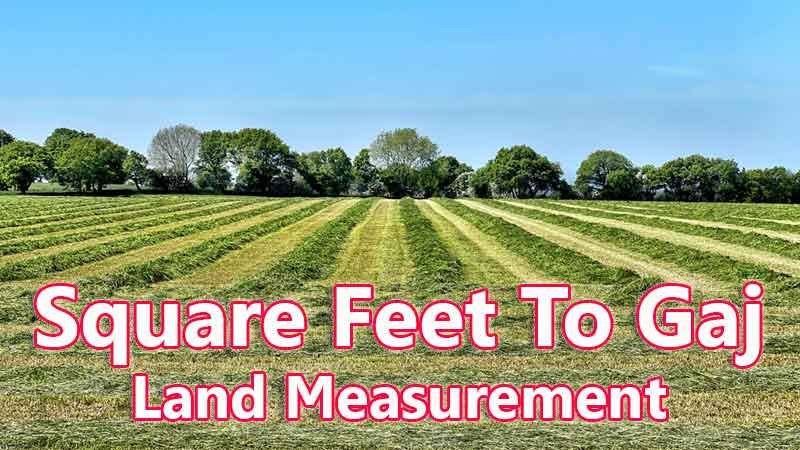# Square Feet To Gaj Converter

Square Feet is a traditional land unit used to measure the land Area standardly. Here we are using the square feet to gaj unit value conversion. And square feet also comes under these land area units like Gonda, Kani, and gaj. In West Bengal, One square feet is directly equivalent to 0.112188 gaj.

Input Here

square feet

Output

gaj

1 square feet = 0.112188 gaj

## Measurement

Square Feet means - a unit of land measurement. This tool provides great calculation over square feet to gaj, 1 square feet to gaj, 1 square feet in gaj, 1 square feet equal to and etc. To convert square feet to gaj, then multiply the unit value by 0.112188. Then, 1 square feet * 0.112188 = 0.112188 gaj. Hence, 1 square feet is equal to 0.112188 gaj.

gaj also comes under this land measurement unit and we are comparing values between square feet traditional unit with other side land measurement unit.

## Formula For square feet to gaj Conversion(square feet to gaj formula)

The base formula for this square feet to gaj converter is

gaj = square feet * 0.112188

## How many gaj in a square feet?

Step 1: To Convert 1 square feet to gaj

Step 2: Applying formula gaj = square feet * 0.112188, (i.e) multiply the unit value by 0.112188.

Step 3: Then, gaj = 1 * 0.112188 = 0.112188.

Step 4: Hence, 1 square feet is equal to 0.112188 gaj.Some square feet to gaj Conversion Chart for your reference:

 1 square feet = 0.112188 gaj 2 square feet = 0.224376 gaj 3 square feet = 0.336565 gaj 4 square feet = 0.448753 gaj 5 square feet = 0.560941 gaj 6 square feet = 0.673129 gaj 7 square feet = 0.785317 gaj 8 square feet = 0.897506 gaj 9 square feet = 1.00969 gaj 10 square feet = 1.12188 gaj

The square feet to gaj conversion chart is above listed for your reference. This chart, however, represents the simple math calculation involved in the square feet to gaj convertion online.

For Example: How much is 1 square feet to gaj

Solution:

= (square feet * 0.112188)

= (1 x 0.112188)

= 0.112188 gaj

For Example: How much is 120 square feet in gaj

Solution:

= (square feet * 0.112188)

= (120 x 0.112188)

= 13.4626 gaj

This formula provides an instant answer for all your questions / People also search:

• 1 square feet to gaj
• 1 square feet is equal to how many gaj
• Formula to convert square feet to gaj online
• How to convert square feet to gaj?
• How much gaj is equal to a one square feet?
• How many gaj in square feet?
• 1 square feet equal to gaj